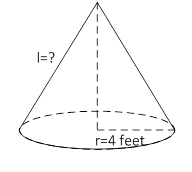### Sample Problem

If the surface area of the cone is 180 feet2, what is the missing length? Use 3.14 for π and round the answer to the nearest hundredth.The missing length is feet.

#### Solution

SA = πr2 + πr × l

r = 4 feet

So, l = (180- 3.14 × 16) ÷ (3.14 × 4)

= 10.33 feet